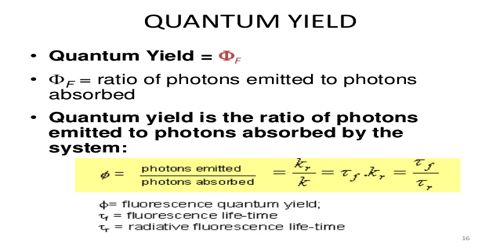# Determination of Quantum Yield

Determination of Quantum Yield

The fluorescence quantum yield is defined as the ratio of the number of photons emitted to the number of photons absorbed. Experimentally, relative fluorescence quantum yields can be determined by measuring fluorescence of a fluorophore of known quantum yield. For experimental determination of Φ, the number of molecules reacting per unit time and the quanta of radiation absorbed per unit time must be known. The first quantity can be measured by a number of ways.

If a gaseous reaction involves a change in volume, manometric methods are most convenient; for reaction of other types either the reactant or the product can be analyzed by physical methods.

The second quantity, i.e., the number of quanta absorbed is determined by measuring the intensity of the monochromatic radiation before and after passing through the system. The intensity can be measured by a thermopile or bolometer. Photoelectric cells also can be used to measure the intensity of radiation. They are most suitable for comparative study. If, however, one or two suitable reactions can be found out whose quantum yields and rates of reaction are accurately known, these reactions can be used to measure the intensity of radiation. Devices which use such reactions are known as actinometers which are frequently used. Since the rates of reaction vary with the wavelength, these reactions must be standardized for different wavelengths. The reduction of HgCl2 by (NH4)2C2O4 in presence of trace quantity of ferric salt, to Hg2Cl2 can be used for measurement of intensity of radiation.

The reaction concerned is

HgCl2 + (NH4)2C2O4 → 2NH4Cl + CO2 + Hg2Cl2

The extent of reaction can be easily measured by weighing the amount of Hg2Cl2 formed. Another reaction commonly used is the decomposition of oxalic acid to carbon dioxide by radiation in presence of uranium salt which acts as a sensitizer. The unreacted oxalic acid is determined by titrating with potassium permanganate alter exposure to the radiation for a definite time. From these data the rate of the absorption of radiation quanta is calculated.